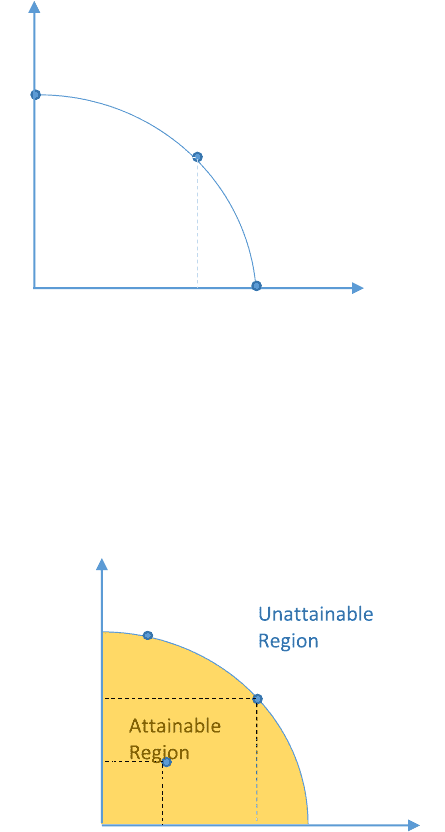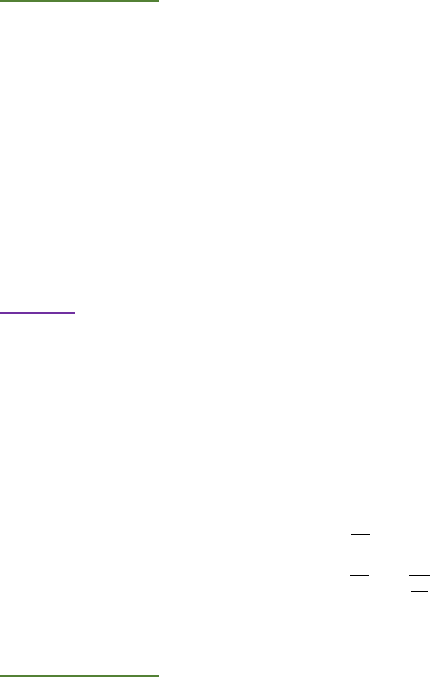# MGEA02H3 Study Guide - Midterm Guide: Yottabyte, Opportunity Cost, Takers

602 views44 pages

University of Toronto Scarborough
MGEA02
Introduction to Microeconomics
Exam Guide
PART I Lecture Key Points Review
PART II Exam Questions & Answers and Explanations
Unlock document

This preview shows pages 1-3 of the document.
Unlock all 44 pages and 3 million more documents.1. What is Economics?
Economics is the study of the use of scarce resources to satisfy unlimited human wants.
2. What is Opportunity Cost?
Value of the alternative foregone (Value of giving up something).
Explicit Costs vs Implicit Costs
Both are parts of opportunity costs. Both are given up when the action is taken.
3. Production Possible Frontier (P.P.F)
P.P.F shows set of output possibilities such that the production of each good is the maximum
possible, given the efficient production of the other good.
Graph I:
A: All resources are used to produce Y
B: All resources are used to produce X
C: Certain amount of resources are used to produce Y, certain amount of resources are used to
produce X
Graph II:
XB
YA
Y
X
Y0
C
B
X0
A
Y
X
YB
C
B
XA
A
Unlock document

This preview shows pages 1-3 of the document.
Unlock all 44 pages and 3 million more documents.1) Economically efficient (Point B)
Inefficient Allocation (Point A)
Therefore, A doesn’t use the maximum resources
2) XB > XA and YB > YA
Therefore, move from A to B has no opportunity cost
Move from B to C will give up some X for more Y, so will has some opportunity cost
3) Attainable Region: Along the P.P.F curve and under the P.P.F curve
Unattainable Region: Outside the P.P.F curve
4) Economically Efficient: Points along the P.P.F curve
Inefficient Allocations: Points within the P.P.F curve
EXAM Questions:
A country produces goods X and Y and has the following equation for its production possibilities frontier
(or production possibilities curve):
Y = 400 2.5X − .025X2
The point X = 40, Y = 250 is:
A) Attainable and efficient B) attainable and inefficient C) unattainable
D) unattainable and efficient E) imaginable and feasible F) unattainable and inefficient
G) none of the above
Put X=40 into the equation, Y = 400 2.5(40) 0.025(40^2) =260
Y = 250 < 260, Thus the point is inside the P.P.F curve, which is attainable and inefficient.
4. Opportunity Cost of X = OCX = - 

Opportunity Cost of Y = OCY = - 
 = - (


EXAM Questions:
A country produces goods X and Y and has the following equation for its production possibilities frontier
(or production possibilities curve):
Y = 400 2.5X − .025X2
Unlock document

This preview shows pages 1-3 of the document.
Unlock all 44 pages and 3 million more documents.